﻿ 一种简易型小功率无线电能传输系统的研究与设计 Study and Design of a Low Power Wireless Power Transfer System

Open Journal of Circuits and Systems
Vol. 07  No. 04 ( 2018 ), Article ID: 28042 , 8 pages
10.12677/OJCS.2018.74016

Study and Design of a Low Power Wireless Power Transfer System

Jian He, Xiaoqing Ou, Yu Wang, Wei Peng, Minsheng Yang*

School of Electrical and Information Engineering, Hunan University of Arts and Sciences, Changde Hunan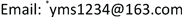Received: Nov. 26th, 2018; accepted: Dec. 10th, 2018; published: Dec. 17th, 2018ABSTRACT

A wireless power charging system is proposed in this paper. Based on the theory of electromagnetism induction, the power transfers from source coil to load coil without metallic contact. The designed system includes the following components: Voltage transformation, rectifier filter, voltage stabilization, PWM, transmission and reception. The designed device could be moved without limit and the design of the circuit is relatively simple and very easy to achieve. As the experimental results show, with an air gap of centimeter level, the transfer efficiency is up to 70%.

Keywords:Wireless Charging, Electromagnetic Induction, Transmission Efficiency1. 引言

2. 基本原理Figure 1. Equivalent circuit diagram of wireless power transmission

3. 硬件设计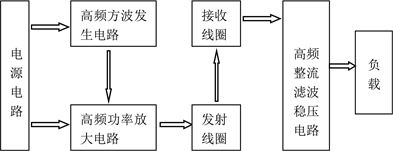Figure 2. Overall design block diagram

3.1. STM32最小应用系统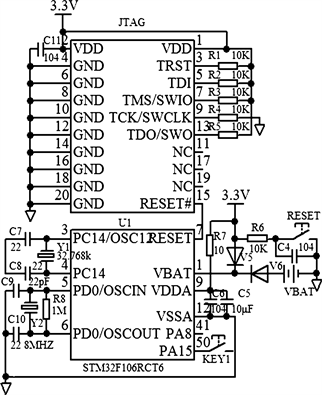Figure 3. Design of minimum system of single chip microcomputer

3.2. 电源电路的设计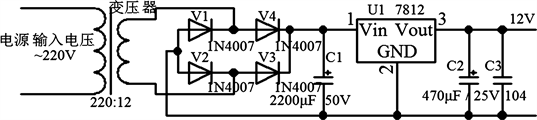Figure 4. Power circuit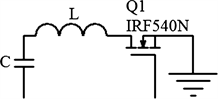Figure 5. Transmitting circuit

3.3. 发射电路的设计

3.4. 接收电路的设计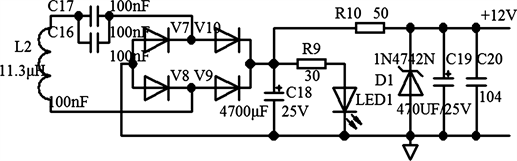Figure 6. Receiving circuit

3.5. 充电电路的设计Figure 7. Charging circuit

4. 软件设计

4.1. 接口定义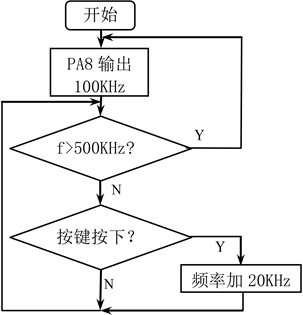Figure 8. Program flow chart

4.2. 程序流程图

5. 实物制作及实验现象

5.1. 检测高频方波

5.2. 实验现象

5.3. 实验数据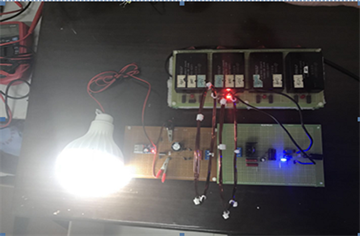Figure 9. Lights 5W energy-saving lamps from a distance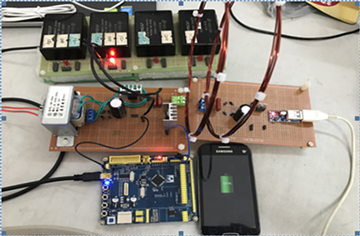Figure 10. Wireless charging of mobile phones

${P}_{in}={U}_{\text{in}}{I}_{\text{in}}$

${P}_{out}={U}_{\text{out}}{I}_{\text{out}}$

$\eta =\frac{{U}_{\text{out}}{I}_{\text{out}}}{{U}_{\text{in}}{I}_{\text{in}}}×100%$

6. 总结

Study and Design of a Low Power Wireless Power Transfer System[J]. 电路与系统, 2018, 07(04): 126-133. https://doi.org/10.12677/OJCS.2018.74016

1. 1. 杨国明. 电磁谐振耦合无线电能传输技术的研究[D]. 沈阳工业大学, 2013.

2. 2. 鲁川. 共振型无线电力传输装置成功点亮2.1 m外的60W灯泡[J]. 华东电力, 2007(6): 97-97.

3. 3. 胡新福. 基于电磁感应方式的锂离子电池无线充电器的设计与实现[J]. 西昌学院学报(自然科学版), 2014, 28(1): 61-65.

4. 4. 汪都. 高频小功率无线充电技术研究[D]. 哈尔滨工业大学, 2016.

5. 5. 王红亮, 张天文, 符多铎, 张世界. 无线充电系统的设计与实现[J]. 火力与指挥控制, 2014, 39(11): 183-186.

6. 6. 施建喆. 电磁感应与无线充电[J]. 科技风, 2016(21): 125.

7. 7. 潘力. 一种无线电能传输系统的设计[J]. 大众科技, 2013(3): 84-86.

8. 8. 付文莉. 直流稳压电源整流电路工作原理浅述[J]. 科技创新与应用, 2013(13): 147-148.

NOTES

*通讯作者。